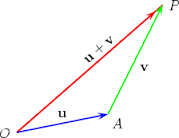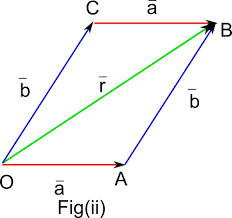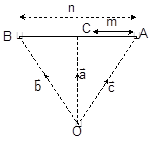or# RD Sharma Class 12 and Chapter Algebra Of Vectors Maths Solutions

## Maths Class 12 and Chapter Algebra Of Vectors RD Sharma SolutionsFilter
Filters :
CLASSES
•
•
•
•
•
•
•
CHAPTERS
•
• 24 More

### RD SHARMA Class 12 | ALGEBRA OF VECTORS

Prove that a necessary and sufficient condition for three vectors ` vec a , vec b` and ` vec c` to be coplanar is that there exist scalars `l , m , n` not all zero simultaneously such that `l vec a+m vec b+n vec c= vec0dot`### RD SHARMA Class 12 | ALGEBRA OF VECTORS

Prove that the following vectors are non-coplanar: `3 hat i+ hat j- hat k ,2 hat i- hat j+7 hat k` and `7 hat i- hat j+23 hat k` ` hat i+2 hat j+3 hat k ,2 hat i+ hat j+3` and ` hat i+ hat j+ hat k`According to CBSE curriculum, the students have to follow the NCERT books throughout their academic life. However, the same books are seemed to be efficient till class 7th due to the limited information they contain.

The Maths NCERT books comprise of basic theory, general problems and selective formulas, which altogether make it quite hard for students to learn beyond the syllabus. Studying maths from books does not suffice the learning appetite of a student required to excel in board exams.

For the thorough understanding of mathematical concepts, the class 12 students must refer to the RD Sharma book. The RD Sharma book contains solved examples to strengthen your base and brush up your concepts.  However, the RD Sharma book also contains several unsolved questions for effective practice and the students wander here and there for the solutions of those unsolved questions.  For that purpose, Doubtnut has really been a boon for the class 12 students.  Doubtnut provides the RD Sharma solutions in video tutorials to students for better understanding and swift revision as well.

## INTRODUCTION

• The magnitude of the vector (a,b,c) is defined as W=  √a2+b2+c2
• A vector can be divided by its own length to convert it into a unit vector, i.e.? = u / |u|. (The vectors have been denoted by bold letters.)
• If the coordinates of point A are xA, yA, zA and of point B are xB, yB, zB then the vector that connects point A to point B is given by the vector( r), where r = (xB - xA)i + (yB – yA) j + (zB – zA)k , here i, j and k symbolize the unit vector along x, y and z axis respectively.

## Some key points of vectors

1) The magnitude of a vector is a scalar quantity

2) Vectors can be multiplied by a scalar. The result is another vector.

3) Suppose c is a scalar quantity and = (a, b) is a vector quantity, then the scalar multiplication is defined by cv = c (a, b) = (ca, cb). Hence each component of the vector is multiplied by the scalar and produces another vector.

4) If two vectors are of the same magnitude then they can be added or subtracted from each other. The result is again a vector.

• If u, v and w are three vectors and c, d are two scalars then the following results of vector addition hold true:

1) (u+v=v+u) it follows the commutative law of addition.

2) u+0=u

3) u+(-u)=0 it shows the existence of additive inverses.

4) c (du)=(cd)u

5) (c+d)u=cu+du

6) c(u+v)=cu+cv

7) 1(u)=u

8) u+(v+w)=(u+v)+w, it follows the associative law of addition.

• Given two vectors u and v, their sum is written as (u+v) is also a vector obtained by first bringing the initial point of v to the terminal point of u and then connecting the initial point of u to the terminal point of v giving a uniform direction by completing the triangle OAP. This is called Triangle Law of Addition.• Suppose a and b be the two vectors. From the initial point of a, vector b is drawn and the parallelogram AOCB is completed with OA and OB as adjacent sides. The vector OC is termed as the sum of a and b. This is known as the parallelogram law of vectors.Polygon Law of Addition is used for adding more than two vectors.

## Internal and External Division

1) If A and B are two points with position vectors a and b respectively and C is the point which divides AB internally in the ratio m: n, then the position vector of C is written asOC = (mb + na)/ (m + n). This is called an internal division.

2) If A and B are two points with position vectors a and b respectively and C is the point which divides AB externally in the ratio m: n, then the position vector of C is written as OC = (mb - na)/ (m-n)3) In case, C is the mid-point of AB, then the position vector of C is (a + b)/2.

4) The position vector of point C on AB can be assumed as c = la + mb, where l + m = 1.

• If circumcentre is the origin and position vectors a, b and c are the vertices of the triangle then the position vector of orthocentre will be -(a+b+c).
• a.b is the product of the magnitude of one vector and magnitude of the projection of the other vector in the direction of former.
• a.b= |a| Projection of |b| in the direction of a= |b|. Projection of |a| in the direction of b.

## Some Basic Rules of Algebra of Vectors

1) a.a = |a|2 = a2

2) a.b = b.a

3) a.0 = 0

4) a.b = (a cos q)b = (projection of a on b)b = (projection of b on a) a

5) a.(b + c) = a.b + a.c (This is also termed as the distributive law)

6) (la).(mb) = lm (a.b)

7) (a ± b)2 = (a ± b) . (a ± b) = a2 + b2 ± 2a.b

8) If a and b are non-zero vectors, then the angle between them is written as cos θ = a.b/|a||b|

9) a x a = 0

10) (axb)=-(bxa)

11) a x (b + c) = a x b + a x c

• Any vector perpendicular to the plane a and b is l(a x b) where l is a real number.
• Unit vector perpendicular to the plane a and b is ± (a x b)/ |a x b|
• The position of cross(x) and dot(•) can be interchanged without altering the product. Hence it is also represented by [a b c]

1) [abc]=[bca]=[cab]

2) [abc]=-[bac]

3) [ka b c] = k[a b c]

4) [a+b c d] = [a c d] + [b c d]

5) ax(bxc)=(axb)xc, if some or all a, b and c are zero vectors or a and c are collinear.

## Methods to prove collinearity of vectors

1) Two vectors a and b are termed as collinear if there exists k? R such that a = kb.

2) If pxq=0, then p and q are collinear in nature.

3) Three vectors A(a), B(b) and C(c) are collinear if there exists k? R such that AB = kBC i.e. b-a = k (c-b).

4) If (b-a) x (c-b) = 0, then A, B and C are collinear.

5) A(a), B(b) and C(c) are collinear if scalars are present l, m and n (not all zero) such that la + mb+ nc = 0, where l + m + n = o

• P, q and r are three coplanar vectors if there exists l, m. R such that r = lp + mq i.e., one can be declared as a linear combination of the other two.
• If [p q r] = 0, then p, q and r are coplanar.
• A(a), B(b), C(c) and D(d)  are the four points lie in the same plane if there exist l, m ? R such that b-a = l(c-b) + m(d-c).
• If [b-a c-b d-c] = 0 then A, B, C, D are coplanar.
• Two lines in space can be parallel, anti-parallel or neither (called skew lines). Let r = a1 + μb1  and r = a+ μb2 are the two lines.
• They intersect if (b1 x b2)(a2 - a1) = 0
• If b1 and bare collinear, then the two lines are parallel.

• The angle between the two planes are the angle between their normal unit vectors i.e. cos q = n1 . n2
• If a, b and c are three coplanar vectors, then the system of vectors a', b' and c' is called  the reciprocal system of vectors if aa' = bb' = cc' = 1 where a' = (b xc) /[a b c] , b' = (c xa)/ [a b c] and c' = (a x b)/[a b c] Also, [a' b' c'] = 1/ [a b c]
• Dot Product of two vectors a and b defined by a = [a1, a2, ..., an] and b = [b1, b2, ..., bn] is given by a1b1  + a2b2 + ...,  + anbn .

## Some Basic Results of Vector Calculus

1) Vectors in the same direction can be added by simply adding their lengths or magnitude. But if the vectors to be added in opposite directions, then their length or magnitude are subtracted and not added.

2) Column vectors are added by only adding their values in each row.

3) You can find the magnitude or length of a vector in three dimensions by using the formula a2 = b2 + c2 + d2, where a is the magnitude of the vector, and b, c, and d are units in each direction.

4) If l1a+ m1b= l2a+ m2b, then l1= l2 and m1= m2

5) Collinear Vectors are also parallel vectors the only difference is that they lie on the same line.

6) When two vectors are parallel, then their dot product is 1 and the cross product of the vector is zero.

7) Two collinear vectors are always linearly dependent.

8) Two non-collinear non-zero vectors are always independent linearly.

9) Three coplanar vectors are always linearly dependent.

10) Three non-coplanar non-zero vectors are always independent linearly.

11) More than three vectors are always linearly dependent.

12) Three vectors are linearly dependent if they are coplanar that means any one of them can be shown as a linear combination of the other two.

## IMPORTANCE OF RD SHARMA MATHEMATICS BOOK CLASS 12

1. Normally the discovering candidates like to rehearse through the RD Sharma book on account of its huge amount of solicitation to get a handle on that enables them to hint at progress for last CBSE comparably as other focused tests.

3. When it comes to science, positive cures and practice is a must. Despite how canny you are or, how mind blowing you are with estimations without explaining various sorts of solicitation, every one of your endeavours is squandered. In this way, it's progressively splendid to go with RD Sharma book.

4. RD Sharma books for class 12 have different planning questions and activities to explain.

5. Conceptualized and gathered by direction zone aces, these books are made by the present test plans. The latest edition of the RD Sharma book contains all the nonstop changes and are twofold checked by experienced instructors.

6. As the book includes both, solved and unsolved problems, the hopefuls can locate the courses of action of such practice questions online at Doubtnut.com.